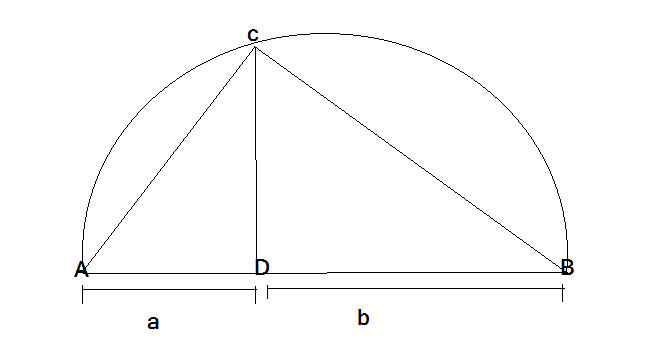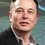# A wonderful Proof of $A.M \geq G.M$ inequalityLets us consider a semicircle with diameter AB. If C is any point on the semicircle, then ABC forms a right angled triangle with right angle at C .

Let CD be the perpendicular to AB dropped from C . Let AD= a and DB=b

The trianges DAC and DCB are similar , hence

$\frac{DA}{DC} = \frac{DC}{DB}$

therefore

$DC^{2} = DA . DB = ab$

and thus $DC = \sqrt{ab}$

if r is the radius of the semicircle , then $DC \leq r$

we know $r = \frac{diameter}{2} = \frac{a + b}{2}$

Thus

$\boxed{ \frac{a + b}{2} \geq \sqrt{ab}}$

If $DC = r$ that is if the Point D comes on the center of the circle then $a = b$

Please reshare if you liked itNote by U Z
6 years, 9 months ago

This discussion board is a place to discuss our Daily Challenges and the math and science related to those challenges. Explanations are more than just a solution — they should explain the steps and thinking strategies that you used to obtain the solution. Comments should further the discussion of math and science.

When posting on Brilliant:

• Use the emojis to react to an explanation, whether you're congratulating a job well done , or just really confused .
• Ask specific questions about the challenge or the steps in somebody's explanation. Well-posed questions can add a lot to the discussion, but posting "I don't understand!" doesn't help anyone.
• Try to contribute something new to the discussion, whether it is an extension, generalization or other idea related to the challenge.

MarkdownAppears as
*italics* or _italics_ italics
**bold** or __bold__ bold
- bulleted- list
• bulleted
• list
1. numbered2. list
1. numbered
2. list
Note: you must add a full line of space before and after lists for them to show up correctly
paragraph 1paragraph 2

paragraph 1

paragraph 2

[example link](https://brilliant.org)example link
> This is a quote
This is a quote
    # I indented these lines
# 4 spaces, and now they show
# up as a code block.

print "hello world"
# I indented these lines
# 4 spaces, and now they show
# up as a code block.

print "hello world"
MathAppears as
Remember to wrap math in $$ ... $$ or $ ... $ to ensure proper formatting.
2 \times 3 $2 \times 3$
2^{34} $2^{34}$
a_{i-1} $a_{i-1}$
\frac{2}{3} $\frac{2}{3}$
\sqrt{2} $\sqrt{2}$
\sum_{i=1}^3 $\sum_{i=1}^3$
\sin \theta $\sin \theta$
\boxed{123} $\boxed{123}$

Sort by:

Geometry proves AM-GM. That's the beauty of mathematics. Reshared!

- 6 years, 9 months ago

Great @megh choksi Keep it up bro

- 6 years, 9 months ago

How to mention someone on brilliant?

- 6 years, 9 months ago

Just write @Name which You want to post

- 6 years, 9 months ago

Geometry can also prove RMS, AM, GM, HM too. Look at here.

- 6 years, 9 months ago

the only condition when DC = r will be when D is the centre of circle. And taking D as the centre of circle will it self make AD = a = radius = b = DB, why do we even need such a long calculation?

- 6 years, 9 months ago